Function Repository Resource:

# RiemannSphereComplexPlot

A 3D rotatable Riemann sphere version of ComplexPlot

Contributed by: Jan Mangaldan
 ResourceFunction["RiemannSphereComplexPlot"][f,z] generates a plot of Arg[f] over the Riemann sphere.

## Details and Options

ResourceFunction["RiemannSphereComplexPlot"] has the attribute HoldAll.
The Riemann sphere is a stereographic projection of the extended complex plane onto a sphere that includes the point at infinity.
ResourceFunction["RiemannSphereComplexPlot"] treats the variable z as local, effectively using Block.
ResourceFunction["RiemannSphereComplexPlot"] uses a cyclic color function over Arg[f] to identify features such as zeros, poles and essential singularities. The color function goes from -π to π counterclockwise around zeros, clockwise around poles and infinite cycles near essential singularities.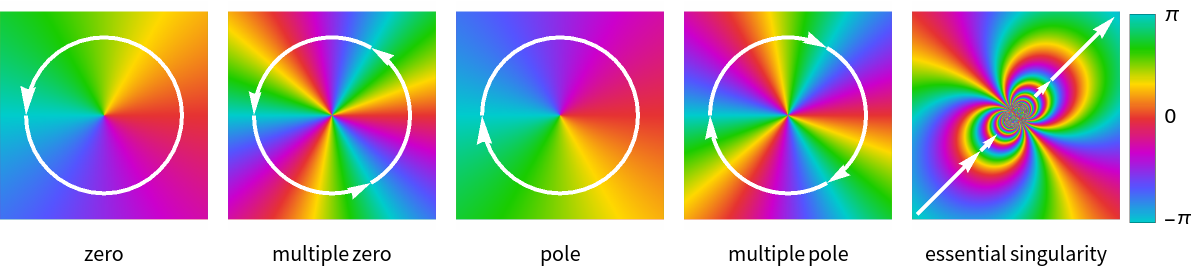No color is applied in any regions where the corresponding f evaluates to None.
Most options for ComplexPlot and SphericalPlot3D are supported.

## Examples

### Basic Examples (3)

Plot a complex function with a zero at 1 and poles at (-1)±2/3:

 In:=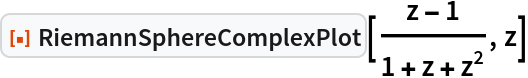Out=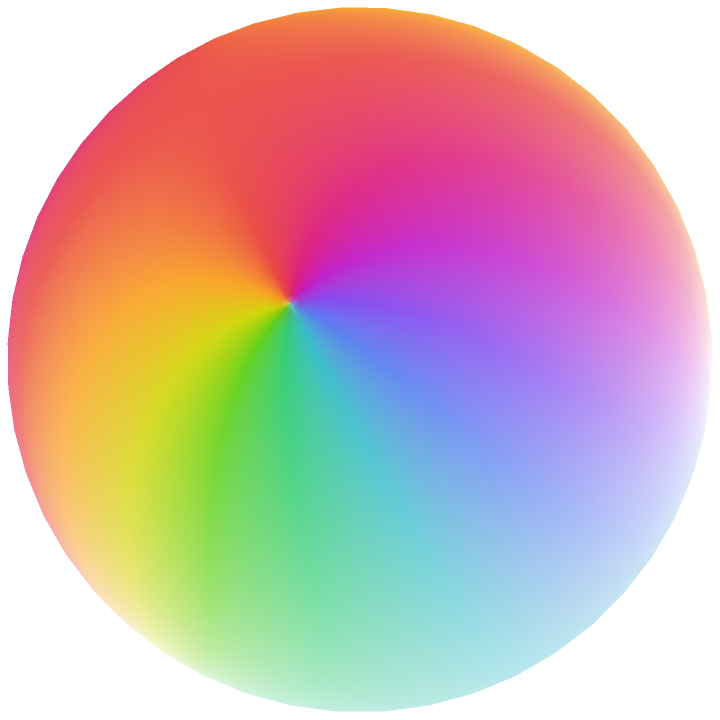Specify a custom ColorFunction and color shading:

 In:=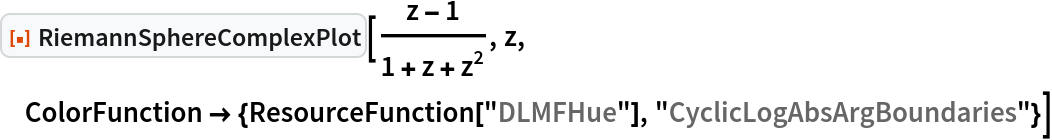Out=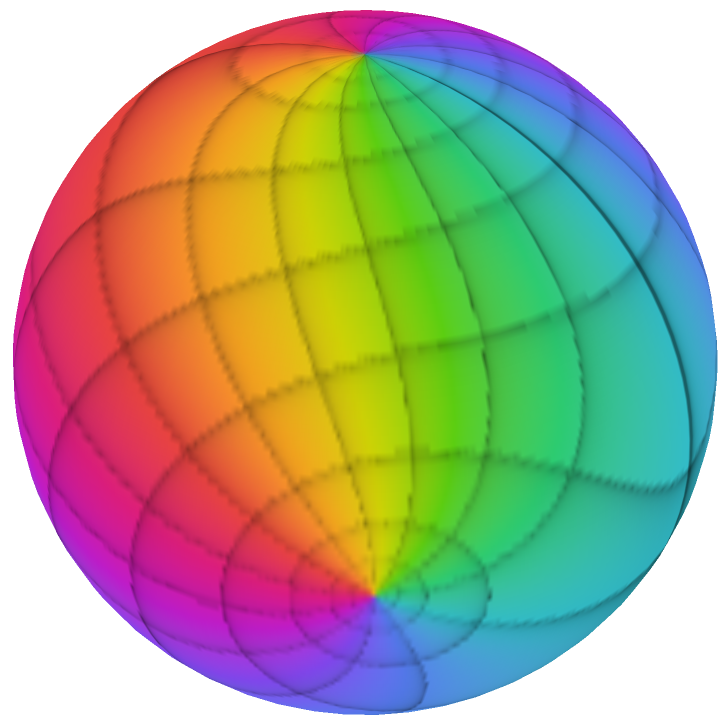Include a legend showing how the colors vary from -π to π:

 In:=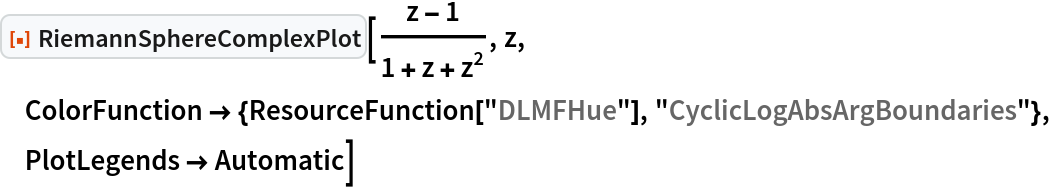Out=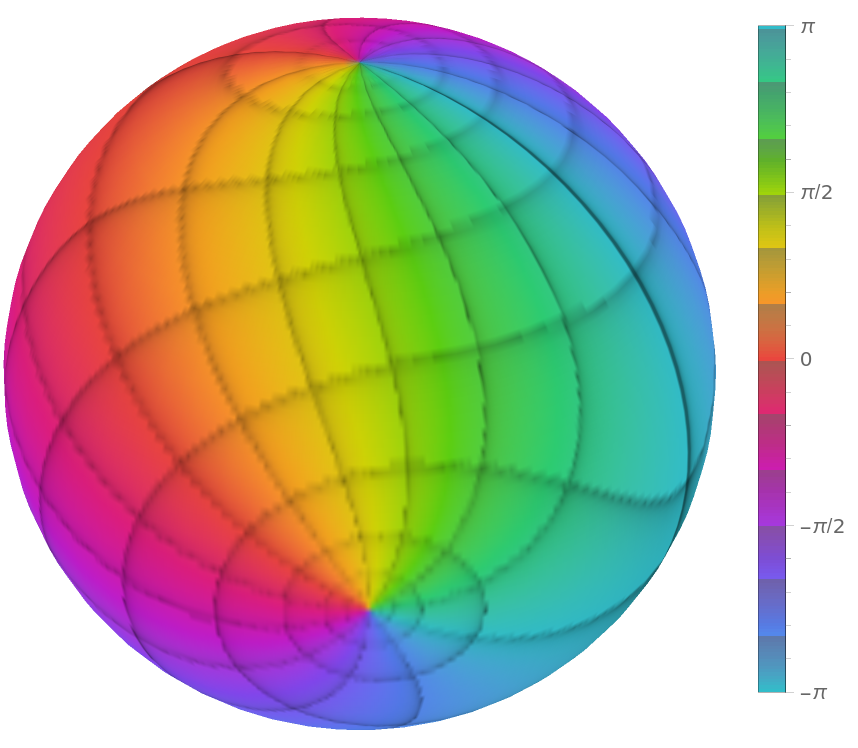### Scope (1)

For functions with a branch cut, RiemannSphereComplexPlot will display a discontinuity in color:

 In:=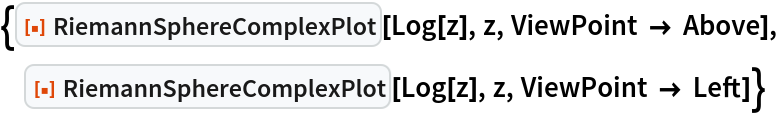Out=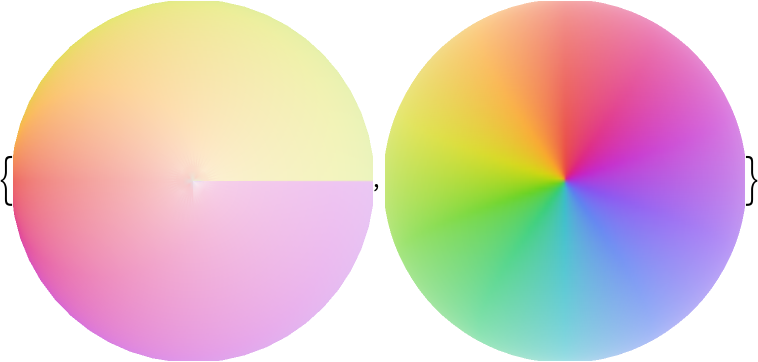### Options (1)

Most options for ComplexPlot and SphericalPlot3D are supported:

 In:=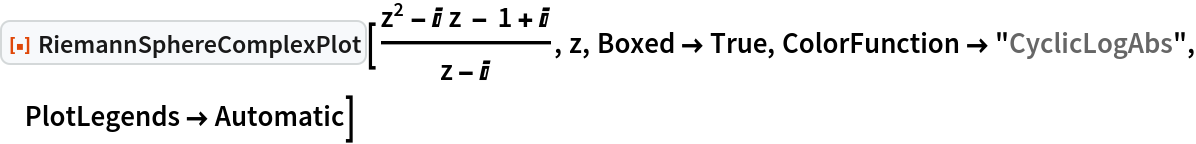Out=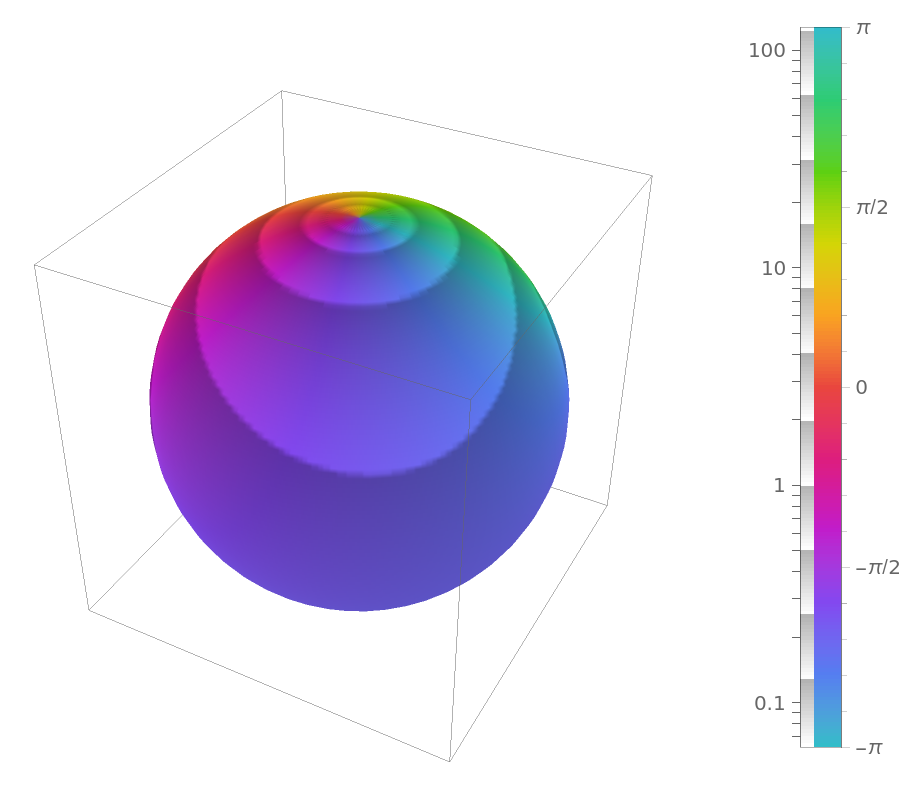### Properties and Relations (1)

In RiemannSphereComplexPlot, the origin is projected to the South Pole of the sphere, and the point at infinity is mapped to the North Pole:

 In:=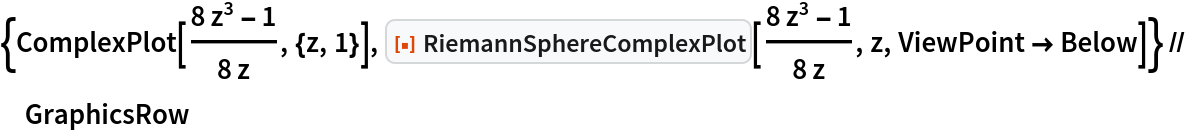Out=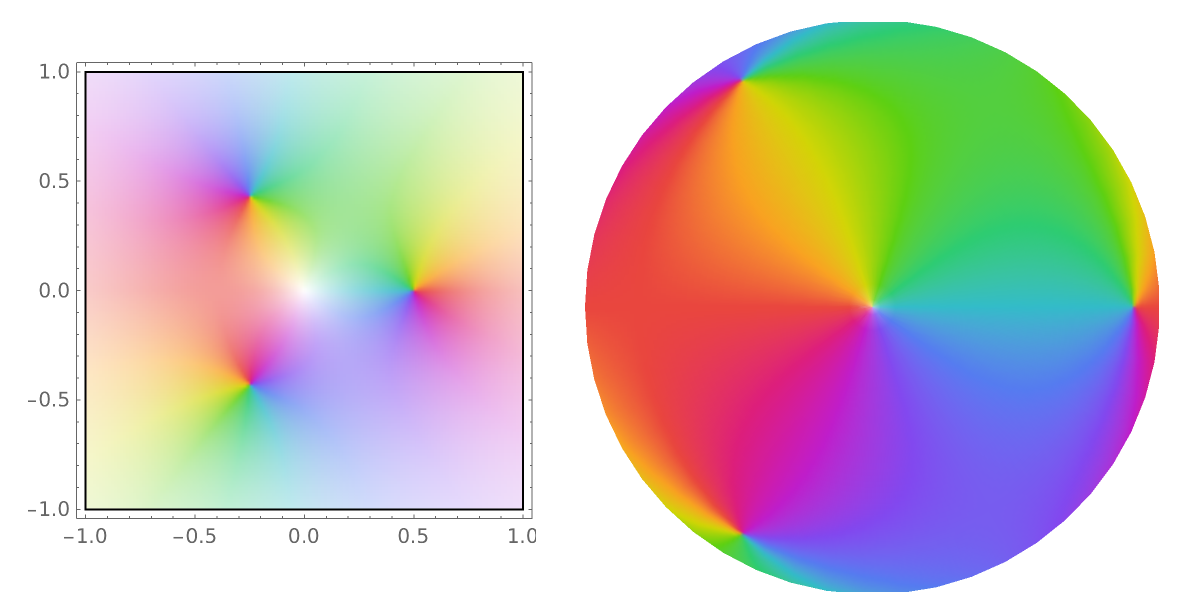### Neat Examples (1)

Use resource function SpinShow to create a spinning Riemann sphere visualization:

 In:=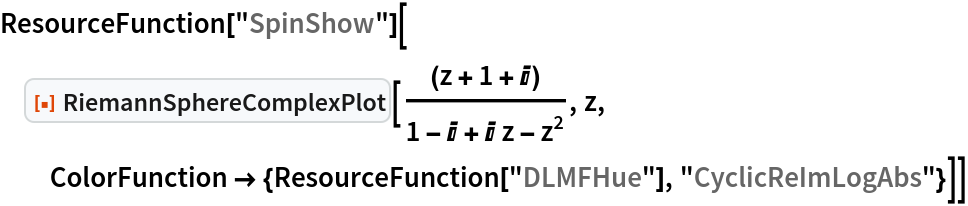Out=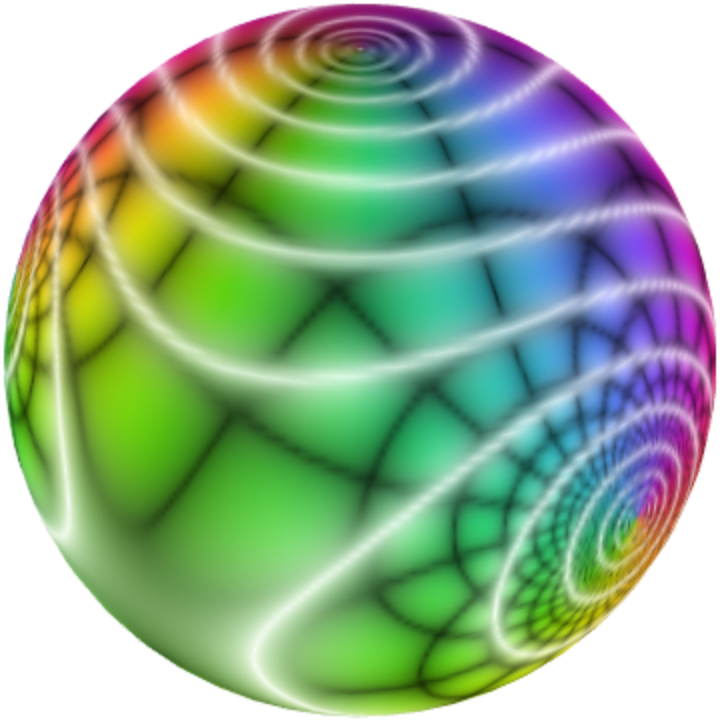## Version History

• 1.0.0 – 15 January 2021